# Machine Learning: Introduction to Neural Networks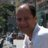Innovation - TILAB - Service Platforms Innovation - "Smart Cities" at Telecom Italia em Telecom Italia
20 de Dec de 2013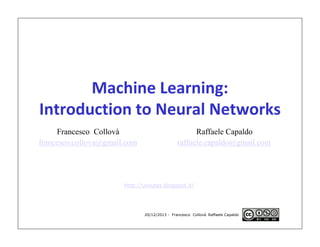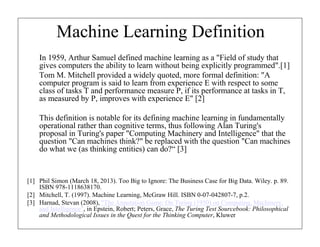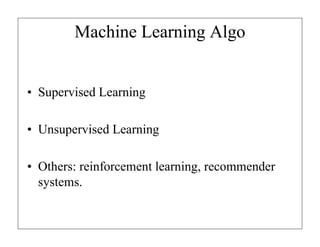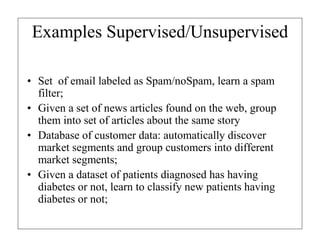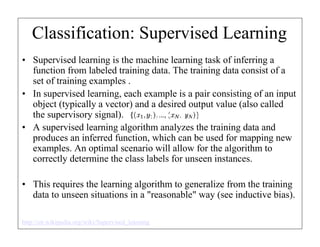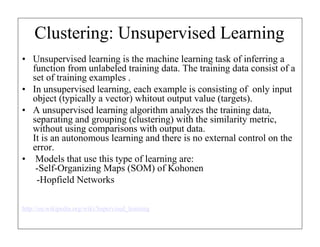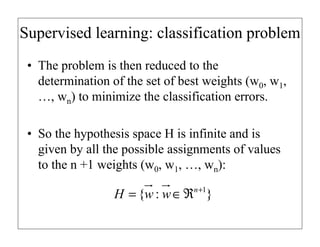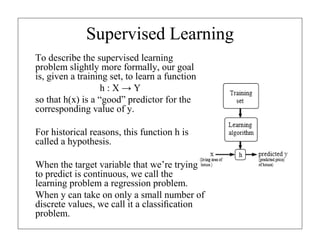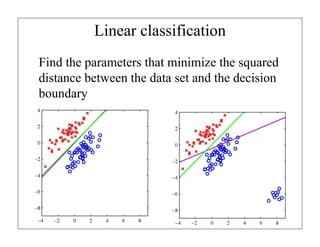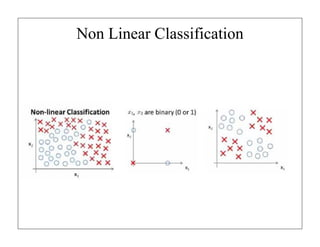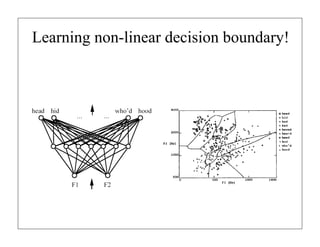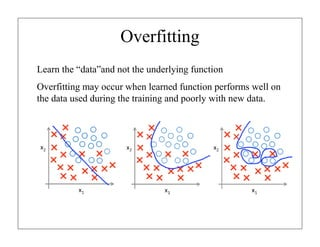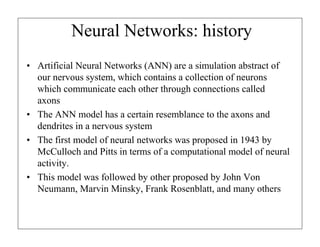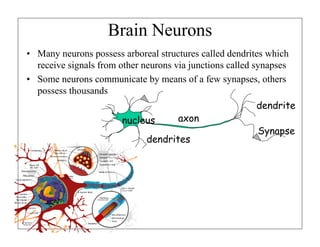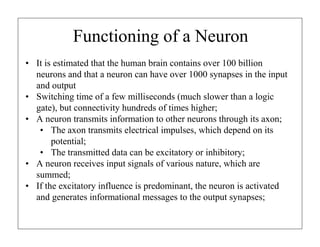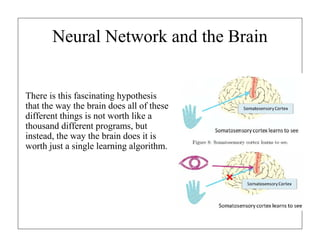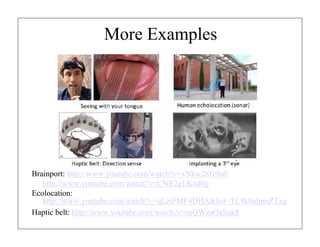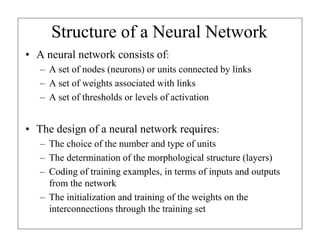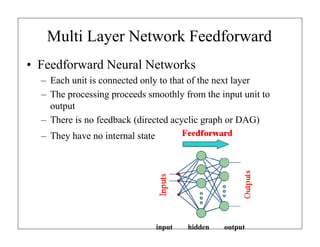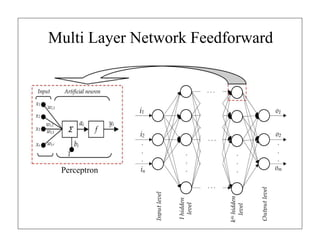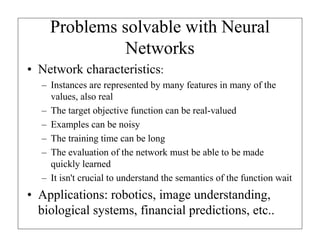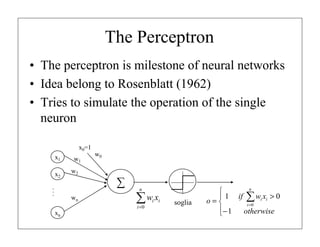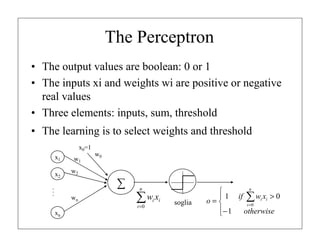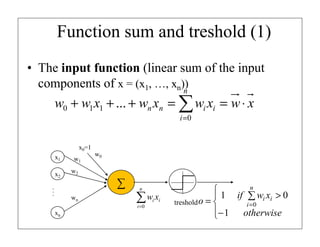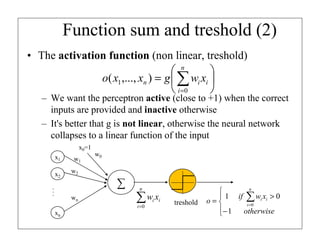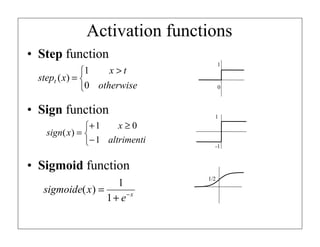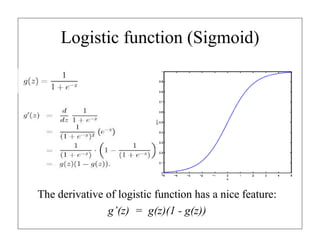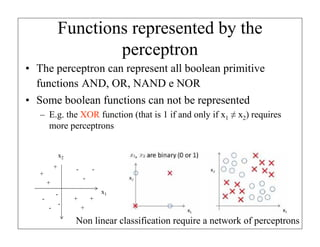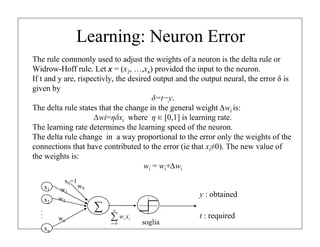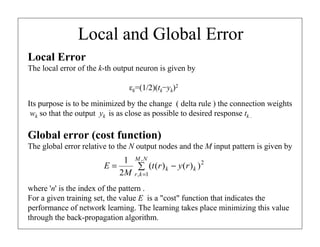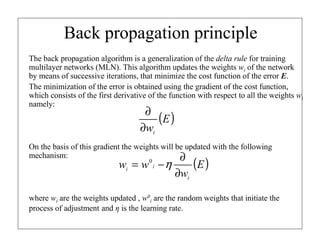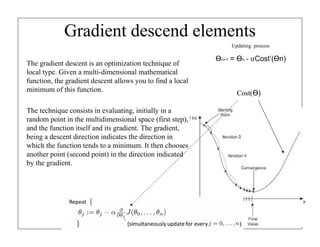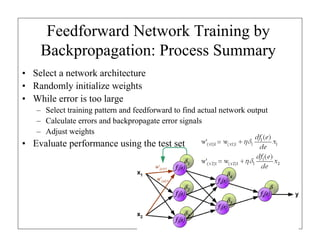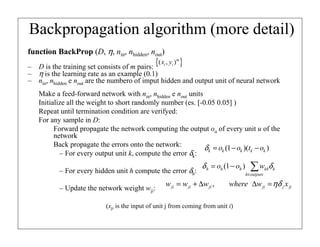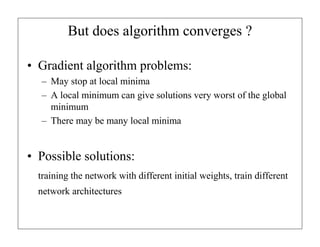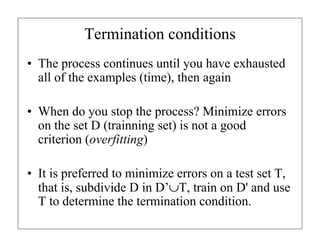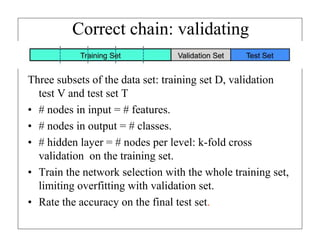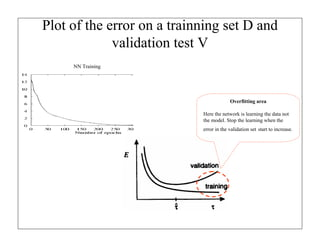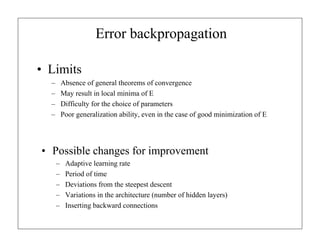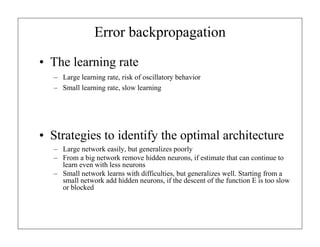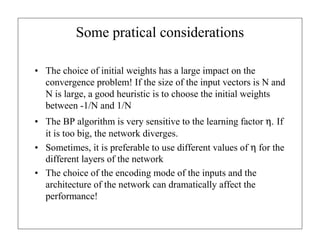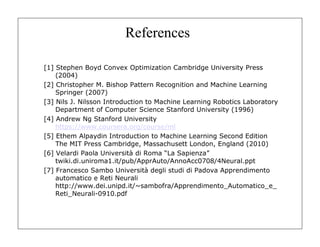1 de 42

### Machine Learning: Introduction to Neural Networks

• 1. Machine Learning: Introduction to Neural Networks Francesco Collovà francesco.collova@gmail.com Raffaele Capaldo raffaele.capaldo@gmail.com http://uroutes.blogspot.it/ 20/12/2013 - Francesco Collovà Raffaele Capaldo
• 2. Machine Learning Definition In 1959, Arthur Samuel defined machine learning as a "Field of study that gives computers the ability to learn without being explicitly programmed". Tom M. Mitchell provided a widely quoted, more formal definition: "A computer program is said to learn from experience E with respect to some class of tasks T and performance measure P, if its performance at tasks in T, as measured by P, improves with experience E"  This definition is notable for its defining machine learning in fundamentally operational rather than cognitive terms, thus following Alan Turing's proposal in Turing's paper "Computing Machinery and Intelligence" that the question "Can machines think?" be replaced with the question "Can machines do what we (as thinking entities) can do?“   Phil Simon (March 18, 2013). Too Big to Ignore: The Business Case for Big Data. Wiley. p. 89. ISBN 978-1118638170.  Mitchell, T. (1997). Machine Learning, McGraw Hill. ISBN 0-07-042807-7, p.2.  Harnad, Stevan (2008), "The Annotation Game: On Turing (1950) on Computing, Machinery, and Intelligence", in Epstein, Robert; Peters, Grace, The Turing Test Sourcebook: Philosophical and Methodological Issues in the Quest for the Thinking Computer, Kluwer
• 3. Machine Learning Algo • Supervised Learning • Unsupervised Learning • Others: reinforcement learning, recommender systems.
• 4. Examples Supervised/Unsupervised • Set of email labeled as Spam/noSpam, learn a spam filter; • Given a set of news articles found on the web, group them into set of articles about the same story • Database of customer data: automatically discover market segments and group customers into different market segments; • Given a dataset of patients diagnosed has having diabetes or not, learn to classify new patients having diabetes or not;
• 5. Classification: Supervised Learning • Supervised learning is the machine learning task of inferring a function from labeled training data. The training data consist of a set of training examples . • In supervised learning, each example is a pair consisting of an input object (typically a vector) and a desired output value (also called the supervisory signal). • A supervised learning algorithm analyzes the training data and produces an inferred function, which can be used for mapping new examples. An optimal scenario will allow for the algorithm to correctly determine the class labels for unseen instances. • This requires the learning algorithm to generalize from the training data to unseen situations in a "reasonable" way (see inductive bias). http://en.wikipedia.org/wiki/Supervised_learning
• 6. Clustering: Unsupervised Learning • Unsupervised learning is the machine learning task of inferring a function from unlabeled training data. The training data consist of a set of training examples . • In unsupervised learning, each example is consisting of only input object (typically a vector) whitout output value (targets). • A unsupervised learning algorithm analyzes the training data, separating and grouping (clustering) with the similarity metric, without using comparisons with output data. It is an autonomous learning and there is no external control on the error. • Models that use this type of learning are: -Self-Organizing Maps (SOM) of Kohonen -Hopfield Networks http://en.wikipedia.org/wiki/Supervised_learning
• 7. Supervised learning: classification problem • The problem is then reduced to the determination of the set of best weights (w0, w1, …, wn) to minimize the classification errors. • So the hypothesis space H is infinite and is given by all the possible assignments of values to the n +1 weights (w0, w1, …, wn): n +1 H = {w : w ∈ ℜ }
• 8. Supervised Learning To describe the supervised learning problem slightly more formally, our goal is, given a training set, to learn a function h:X→Y so that h(x) is a “good” predictor for the corresponding value of y. For historical reasons, this function h is called a hypothesis. When the target variable that we’re trying to predict is continuous, we call the learning problem a regression problem. When y can take on only a small number of discrete values, we call it a classiﬁcation problem.
• 9. Linear classification Find the parameters that minimize the squared distance between the data set and the decision boundary
• 12. Overfitting Learn the “data”and not the underlying function Overfitting may occur when learned function performs well on the data used during the training and poorly with new data.
• 13. Neural Networks: history • Artificial Neural Networks (ANN) are a simulation abstract of our nervous system, which contains a collection of neurons which communicate each other through connections called axons • The ANN model has a certain resemblance to the axons and dendrites in a nervous system • The first model of neural networks was proposed in 1943 by McCulloch and Pitts in terms of a computational model of neural activity. • This model was followed by other proposed by John Von Neumann, Marvin Minsky, Frank Rosenblatt, and many others
• 14. Brain Neurons • Many neurons possess arboreal structures called dendrites which receive signals from other neurons via junctions called synapses • Some neurons communicate by means of a few synapses, others possess thousands dendrite nucleus axon dendrites Synapse
• 15. Functioning of a Neuron • It is estimated that the human brain contains over 100 billion neurons and that a neuron can have over 1000 synapses in the input and output • Switching time of a few milliseconds (much slower than a logic gate), but connectivity hundreds of times higher; • A neuron transmits information to other neurons through its axon; • The axon transmits electrical impulses, which depend on its potential; • The transmitted data can be excitatory or inhibitory; • A neuron receives input signals of various nature, which are summed; • If the excitatory influence is predominant, the neuron is activated and generates informational messages to the output synapses;
• 16. Neural Network and the Brain There is this fascinating hypothesis that the way the brain does all of these different things is not worth like a thousand different programs, but instead, the way the brain does it is worth just a single learning algorithm.
• 18. Structure of a Neural Network • A neural network consists of: – A set of nodes (neurons) or units connected by links – A set of weights associated with links – A set of thresholds or levels of activation • The design of a neural network requires: – The choice of the number and type of units – The determination of the morphological structure (layers) – Coding of training examples, in terms of inputs and outputs from the network – The initialization and training of the weights on the interconnections through the training set
• 19. Multi Layer Network Feedforward • Feedforward Neural Networks – Each unit is connected only to that of the next layer – The processing proceeds smoothly from the input unit to output – There is no feedback (directed acyclic graph or DAG) – They have no internal state input hidden output
• 20. Multi Layer Network Feedforward Perceptron
• 21. Problems solvable with Neural Networks • Network characteristics: – Instances are represented by many features in many of the values, also real – The target objective function can be real-valued – Examples can be noisy – The training time can be long – The evaluation of the network must be able to be made quickly learned – It isn't crucial to understand the semantics of the function wait • Applications: robotics, image understanding, biological systems, financial predictions, etc..
• 22. The Perceptron • The perceptron is milestone of neural networks • Idea belong to Rosenblatt (1962) • Tries to simulate the operation of the single neuron x0=1 x1 x2 w1 w0 w2 ∑ ... wn n ∑w x i =0 xn i i soglia n   1 if ∑ wi xi > 0 o= i =0 − 1 otherwise 
• 23. The Perceptron • The output values ​are boolean: 0 or 1 • The inputs xi and weights wi are positive or negative real values • Three elements: inputs, sum, threshold • The learning is to select weights and threshold x0=1 x1 x2 w1 w0 w2 ... ∑ wn n ∑w x i =0 xn i i soglia n   1 if ∑ wi xi > 0 o= i =0 − 1 otherwise 
• 24. Function sum and treshold (1) • The input function (linear sum of the input components of x = (x1, …, xn)) n w0 + w1 x1 + ... + wn xn = ∑ wi xi = w ⋅ x i =0 x0=1 x1 x2 w1 w0 w2 ... ∑ wn n ∑w x i =0 xn i i n   1 if ∑ wi xi > 0 treshold o =  i =0 − 1 otherwise 
• 25. Function sum and treshold (2) • The activation function (non linear, treshold)  n  o( x1 ,..., xn ) = g  ∑ wi xi   i =0  – We want the perceptron active (close to +1) when the correct inputs are provided and inactive otherwise – It's better that g is not linear, otherwise the neural network collapses to a linear function of the input x0=1 x1 x2 w1 w0 w2 ... ∑ wn n ∑w x i =0 xn i i treshold n   1 if ∑ wi xi > 0 o= i =0 − 1 otherwise 
• 26. Activation functions • Step function 1 x>t 1 stept ( x) =  0 otherwise • Sign function x≥0 + 1 sign( x ) =  − 1 altrimenti 0 1 -1 • Sigmoid function 1 sigmoide( x ) = 1 + e− x 1/2
• 27. Logistic function (Sigmoid) The derivative of logistic function has a nice feature: g’(z) = g(z)(1 - g(z))
• 28. Functions represented by the perceptron • The perceptron can represent all boolean primitive functions AND, OR, NAND e NOR • Some boolean functions can not be represented – E.g. the XOR function (that is 1 if and only if x1 ≠ x2) requires more perceptrons x2 + - + - + - - - - + + x1 + Non linear classification require a network of perceptrons
• 29. Learning: Neuron Error The rule commonly used to adjust the weights of a neuron is the delta rule or Widrow-Hoff rule. Let x = (x1, …,xn) provided the input to the neuron. If t and y are, rispectivly, the desired output and the output neural, the error δ is given by δ=t−y. The delta rule states that the change in the general weight ∆wi is: ∆wi=ηδxi where η ∈ [0,1] is learning rate. The learning rate determines the learning speed of the neuron. The delta rule change in a way proportional to the error only the weights of the connections that have contributed to the error (ie that xi≠0). The new value of the weights is: wi = wi+∆wi x1 x2 ... xn x0=1 w0 w1 w2 wn y : obtained ∑ n ∑w x i=0 i i soglia t : required
• 30. Local and Global Error Local Error The local error of the k-th output neuron is given by εk=(1/2)(tk−yk)2 Its purpose is to be minimized by the change ( delta rule ) the connection weights wk so that the output yk is as close as possible to desired response tk . Global error (cost function) The global error relative to the N output nodes and the M input pattern is given by 1 M ,N 2 E= ∑ (t (r ) k − y (r ) k ) 2M r , k =1 where 'n' is the index of the pattern . For a given training set, the value E is a "cost" function that indicates the performance of network learning. The learning takes place minimizing this value through the back-propagation algorithm.
• 31. Back propagation principle The back propagation algorithm is a generalization of the delta rule for training multilayer networks (MLN). This algorithm updates the weights wi of the network by means of successive iterations, that minimize the cost function of the error E. The minimization of the error is obtained using the gradient of the cost function, which consists of the first derivative of the function with respect to all the weights wi namely: ∂ (E ) ∂wi On the basis of this gradient the weights will be updated with the following mechanism: ∂ wi = w 0 i − η ∂wi (E ) where wi are the weights updated , w0i are the random weights that initiate the process of adjustment and η is the learning rate.
• 32. Gradient descend elements Updating process The gradient descent is an optimization technique of local type. Given a multi-dimensional mathematical function, the gradient descent allows you to find a local minimum of this function. The technique consists in evaluating, initially in a random point in the multidimensional space (first step), and the function itself and its gradient. The gradient, being a descent direction indicates the direction in which the function tends to a minimum. It then chooses another point (second point) in the direction indicated by the gradient. Өn+1 = Өn - ⍺Cost’(Өn) Cost(Ө)
• 33. Feedforward Network Training by Backpropagation: Process Summary • Select a network architecture • Randomly initialize weights • While error is too large – Select training pattern and feedforward to find actual network output – Calculate errors and backpropagate error signals – Adjust weights • Evaluate performance using the test set 33
• 34. Backpropagation algorithm (more detail) function BackProp (D, η, nin, nhidden, nout) {( x , y ) } D is the training set consists of m pairs: m i i – – η is the learning rate as an example (0.1) – nin, nhidden e nout are the numbero of imput hidden and output unit of neural network Make a feed-forward network with nin, nhidden e nout units Initialize all the weight to short randomly number (es. [-0.05 0.05] ) Repeat until termination condition are verifyed: For any sample in D: Forward propagate the network computing the output ou of every unit u of the network Back propagate the errors onto the network: δ k = ok (1 − ok )(tk − ok ) – For every output unit k, compute the error δk: – For every hidden unit h compute the error δh: – Update the network weight wji: δ h = oh (1 − oh ) w ji = w ji + ∆w ji , ∑w k∈outputs δ kh k where ∆w ji = ηδ j x ji (xji is the input of unit j from coming from unit i)
• 35. But does algorithm converges ? • Gradient algorithm problems: – May stop at local minima – A local minimum can give solutions very worst of the global minimum – There may be many local minima • Possible solutions: training the network with different initial weights, train different network architectures
• 36. Termination conditions • The process continues until you have exhausted all of the examples (time), then again • When do you stop the process? Minimize errors on the set D (trainning set) is not a good criterion (overfitting) • It is preferred to minimize errors on a test set T, that is, subdivide D in D’∪T, train on D' and use T to determine the termination condition.
• 37. Correct chain: validating Three subsets of the data set: training set D, validation test V and test set T • # nodes in input = # features. • # nodes in output = # classes. • # hidden layer = # nodes per level: k-fold cross validation on the training set. • Train the network selection with the whole training set, limiting overfitting with validation set. • Rate the accuracy on the final test set.
• 38. Plot of the error on a trainning set D and validation test V NN Training Overfitting area Here the network is learning the data not the model. Stop the learning when the error in the validation set start to increase.
• 39. Error backpropagation • Limits – – – – Absence of general theorems of convergence May result in local minima of E Difficulty for the choice of parameters Poor generalization ability, even in the case of good minimization of E • Possible changes for improvement – – – – – Adaptive learning rate Period of time Deviations from the steepest descent Variations in the architecture (number of hidden layers) Inserting backward connections
• 40. Error backpropagation • The learning rate – Large learning rate, risk of oscillatory behavior – Small learning rate, slow learning • Strategies to identify the optimal architecture – Large network easily, but generalizes poorly – From a big network remove hidden neurons, if estimate that can continue to learn even with less neurons – Small network learns with difficulties, but generalizes well. Starting from a small network add hidden neurons, if the descent of the function E is too slow or blocked
• 41. Some pratical considerations • The choice of initial weights has a large impact on the convergence problem! If the size of the input vectors is N and N is large, a good heuristic is to choose the initial weights between -1/N and 1/N • The BP algorithm is very sensitive to the learning factor η. If it is too big, the network diverges. • Sometimes, it is preferable to use different values of η for the different layers of the network • The choice of the encoding mode of the inputs and the architecture of the network can dramatically affect the performance!
• 42. References  Stephen Boyd Convex Optimization Cambridge University Press (2004)  Christopher M. Bishop Pattern Recognition and Machine Learning Springer (2007)  Nils J. Nilsson Introduction to Machine Learning Robotics Laboratory Department of Computer Science Stanford University (1996)  Andrew Ng Stanford University https://www.coursera.org/course/ml  Ethem Alpaydin Introduction to Machine Learning Second Edition The MIT Press Cambridge, Massachusett London, England (2010)  Velardi Paola Università di Roma “La Sapienza” twiki.di.uniroma1.it/pub/ApprAuto/AnnoAcc0708/4Neural.ppt  Francesco Sambo Università degli studi di Padova Apprendimento automatico e Reti Neurali http://www.dei.unipd.it/~sambofra/Apprendimento_Automatico_e_ Reti_Neurali-0910.pdf